GeeksforGeeks App
Open AppBrowser
Continue

# K-NN Classifier in R Programming

K-Nearest Neighbor or K-NN is a Supervised Non-linear classification algorithm. K-NN is a Non-parametric algorithm i.e it doesn’t make any assumption about underlying data or its distribution. It is one of the simplest and widely used algorithm which depends on it’s k value(Neighbors) and finds it’s applications in many industries like finance industry, healthcare industry etc.

#### Theory

In the KNN algorithm, K specifies the number of neighbors and its algorithm is as follows:

• Choose the number K of neighbor.
• Take the K Nearest Neighbor of unknown data point according to distance.
• Among the K-neighbors, Count the number of data points in each category.
• Assign the new data point to a category, where you counted the most neighbors.

For the Nearest Neighbor classifier, the distance between two points is expressed in the form of Euclidean Distance.

Example:
Consider a dataset containing two features Red and Blue and we classify them. Here K is 5 i.e we are considering 5 neighbors according to Euclidean distance.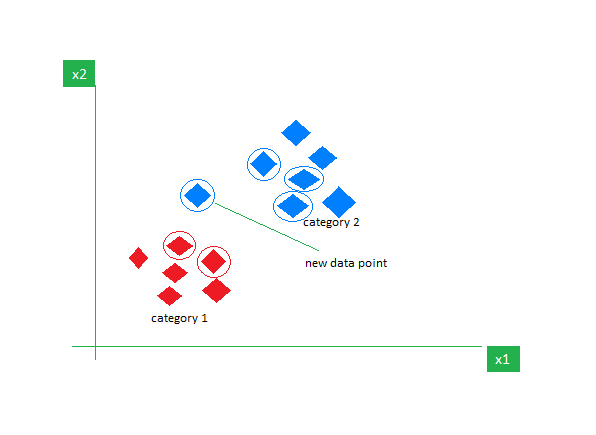So, when a new data point enters, out of 5 neighbors, 3 are Blue and 2 are Red. We assign the new data point to the category with most neighbors i.e Blue.

#### The Dataset

`Iris` dataset consists of 50 samples from each of 3 species of Iris(Iris setosa, Iris virginica, Iris versicolor) and a multivariate dataset introduced by British statistician and biologist Ronald Fisher in his 1936 paper The use of multiple measurements in taxonomic problems. Four features were measured from each sample i.e length and width of the sepals and petals and based on the combination of these four features, Fisher developed a linear discriminant model to distinguish the species from each other.

 `# Loading data``data(iris)``  ` `# Structure ``str``(iris)`#### Performing K Nearest Neighbor on Dataset

Using the K-Nearest Neighbor algorithm on the dataset which includes 11 persons and 6 variables or attributes.

 `# Installing Packages``install.packages(``"e1071"``)``install.packages(``"caTools"``)``install.packages(``"class"``)`` ` `# Loading package``library(e1071)``library(caTools)``library(``class``)`` ` `# Loading data``data(iris)``head(iris)`` ` `# Splitting data into train``# and test data``split <``-` `sample.split(iris, SplitRatio ``=` `0.7``)``train_cl <``-` `subset(iris, split ``=``=` `"TRUE"``)``test_cl <``-` `subset(iris, split ``=``=` `"FALSE"``)`` ` `# Feature Scaling``train_scale <``-` `scale(train_cl[, ``1``:``4``])``test_scale <``-` `scale(test_cl[, ``1``:``4``])`` ` `# Fitting KNN Model ``# to training dataset``classifier_knn <``-` `knn(train ``=` `train_scale,``                      ``test ``=` `test_scale,``                      ``cl ``=` `train_cl\$Species,``                      ``k ``=` `1``)``classifier_knn`` ` `# Confusiin Matrix``cm <``-` `table(test_cl\$Species, classifier_knn)``cm`` ` `# Model Evaluation - Choosing K``# Calculate out of Sample error``misClassError <``-` `mean(classifier_knn !``=` `test_cl\$Species)``print``(paste(``'Accuracy ='``, ``1``-``misClassError))`` ` `# K = 3``classifier_knn <``-` `knn(train ``=` `train_scale,``                      ``test ``=` `test_scale,``                      ``cl ``=` `train_cl\$Species,``                      ``k ``=` `3``)``misClassError <``-` `mean(classifier_knn !``=` `test_cl\$Species)``print``(paste(``'Accuracy ='``, ``1``-``misClassError))`` ` `# K = 5``classifier_knn <``-` `knn(train ``=` `train_scale,``                      ``test ``=` `test_scale,``                      ``cl ``=` `train_cl\$Species,``                      ``k ``=` `5``)``misClassError <``-` `mean(classifier_knn !``=` `test_cl\$Species)``print``(paste(``'Accuracy ='``, ``1``-``misClassError))`` ` `# K = 7``classifier_knn <``-` `knn(train ``=` `train_scale,``                      ``test ``=` `test_scale,``                      ``cl ``=` `train_cl\$Species,``                      ``k ``=` `7``)``misClassError <``-` `mean(classifier_knn !``=` `test_cl\$Species)``print``(paste(``'Accuracy ='``, ``1``-``misClassError))`` ` `# K = 15``classifier_knn <``-` `knn(train ``=` `train_scale,``                      ``test ``=` `test_scale,``                      ``cl ``=` `train_cl\$Species,``                      ``k ``=` `15``)``misClassError <``-` `mean(classifier_knn !``=` `test_cl\$Species)``print``(paste(``'Accuracy ='``, ``1``-``misClassError))`` ` `# K = 19``classifier_knn <``-` `knn(train ``=` `train_scale,``                      ``test ``=` `test_scale,``                      ``cl ``=` `train_cl\$Species,``                      ``k ``=` `19``)``misClassError <``-` `mean(classifier_knn !``=` `test_cl\$Species)``print``(paste(``'Accuracy ='``, ``1``-``misClassError))`

Output:

• Model classifier_knn(k=1):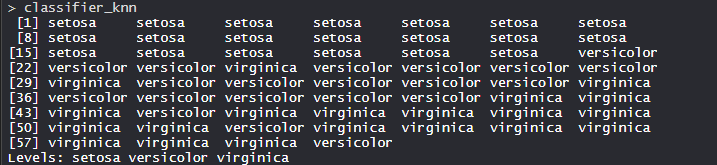The KNN model is fitted with a train, test, and k value. Also, the Classifier Species feature is fitted in the model.

• Confusion Matrix: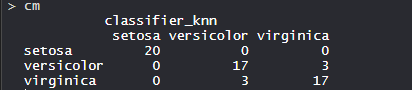So, 20 Setosa are correctly classified as Setosa. Out of 20 Versicolor, 17 Versicolor are correctly classified as Versicolor and 3 are classified as virginica. Out of 20 virginica, 17 virginica are correctly classified as virginica and 3 are classified as Versicolor.

• Model Evaluation:
(k=1)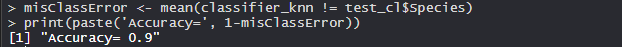The model achieved 90% accuracy with k is 1.

(K=3)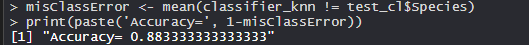The model achieved 88.33% accuracy with k is 3 which is lower than when k was 1.

(K=5)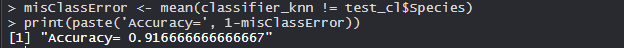The model achieved 91.66% accuracy with k is 5 which is more than when k was 1 and 3.

(K=7)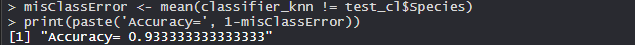The model achieved 93.33% accuracy with k is 7 which is more than when k was 1, 3, and 5.

(K=15)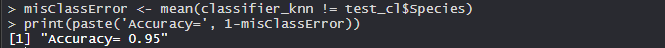The model achieved 95% accuracy with k is 15 which is more than when k was 1, 3, 5, and 7.

(K=19)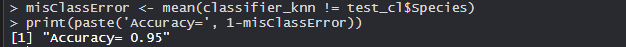The model achieved 95% accuracy with k is 19 which is more than when k was 1, 3, 5, and 7. Its same accuracy when k was 15 which means now increasing k values doesn’t affect the accuracy.

So, K Nearest Neighbor is widely used in the industry.

My Personal Notes arrow_drop_up